# Data analysis and Visualization with Python

Python is a great language for doing data analysis, primarily because of the fantastic ecosystem of data-centric Python packages. Pandas is one of those packages, and makes importing and analyzing data much easier. In this article, I have used Pandas to analyze data on Country Data.csv file from UN public Data Sets of a popular ‘statweb.stanford.edu’ website.
As I have analyzed the Indian Country Data, I have introduced Pandas key concepts as below. Before going through this article, have a rough idea of basics from matplotlib and csv.

Installation
Easiest way to install pandas is to use pip:

`pip install pandas`

Creating A DataFrame in Pandas

Creation of dataframe is done by passing multiple Series into the DataFrame class using pd.Series method. Here, it is passed in the two Series objects, s1 as the first row, and s2 as the second row.
Example:

 `# assigning two series to s1 and s2 ` `s1 ``=` `pd.Series([``1``,``2``]) ` `s2 ``=` `pd.Series([``"Ashish"``, ``"Sid"``]) ` `# framing series objects into data ` `df ``=` `pd.DataFrame([s1,s2]) ` `# show the data frame ` `df ` ` `  `# data framing in another way ` `# taking index and column values ` `dframe ``=` `pd.DataFrame([[``1``,``2``],[``"Ashish"``, ``"Sid"``]], ` `        ``index``=``[``"r1"``, ``"r2"``], ` `        ``columns``=``[``"c1"``, ``"c2"``]) ` `dframe ` ` `  `# framing in another way  ` `# dict-like container ` `dframe ``=` `pd.DataFrame({ ` `        ``"c1"``: [``1``, ``"Ashish"``], ` `        ``"c2"``: [``2``, ``"Sid"``]}) ` `dframe `

Output:

```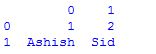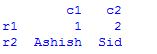```

Importing Data with Pandas

The first step is to read the data. The data is stored as a comma-separated values, or csv, file, where each row is separated by a new line, and each column by a comma (,). In order to be able to work with the data in Python, it is needed to read the csv file into a Pandas DataFrame. A DataFrame is a way to represent and work with tabular data. Tabular data has rows and columns, just like this csv file(Click Download).
Example:

 `# Import the pandas library, renamed as pd ` `import` `pandas as pd ` ` `  `# Read IND_data.csv into a DataFrame, assigned to df ` `df ``=` `pd.read_csv(``"IND_data.csv"``) ` ` `  `# Prints the first 5 rows of a DataFrame as default ` `df.head() ` ` `  `# Prints no. of rows and columns of a DataFrame ` `df.shape `

Output:

```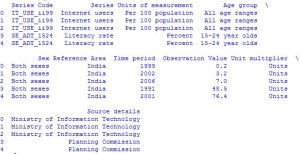```
```29,10
```

Indexing DataFrames with Pandas

Indexing can be possible using the pandas.DataFrame.iloc method. The iloc method allows to retrieve as  many as rows and columns by position.
Examples:

 `# prints first 5 rows and every column which replicates df.head() ` `df.iloc[``0``:``5``,:] ` `# prints entire rows and columns ` `df.iloc[:,:] ` `# prints from 5th rows and first 5 columns ` `df.iloc[``5``:,:``5``] `

Indexing Using Labels in Pandas

Indexing can be worked with labels using the pandas.DataFrame.loc method, which allows to index using labels instead of positions.
Examples:

 `# prints first five rows including 5th index and every columns of df ` `df.loc[``0``:``5``,:] ` `# prints from 5th rows onwards and entire columns ` `df ``=` `df.loc[``5``:,:] `

The above doesn’t actually look much different from df.iloc[0:5,:]. This is because while row labels can take on any values, our row labels match the positions exactly. But column labels can make things much easier when working with data. Example:

 `# Prints the first 5 rows of Time period ` `# value  ` `df.loc[:``5``,``"Time period"``] `

```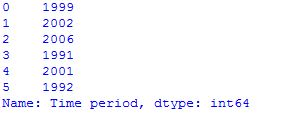```

DataFrame Math with Pandas

Computation of data frames can be done by using Statistical Functions of pandas tools.
Examples:

 `# computes various summary statistics, excluding NaN values ` `df.describe() ` `# for computing correlations ` `df.corr() ` `# computes numerical data ranks ` `df.rank() `

```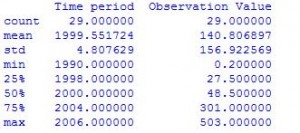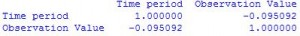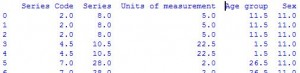```

Pandas Plotting

Plots in these examples are made using standard convention for referencing the matplotlib API which provides the basics in pandas to easily create decent looking plots.
Examples:

 `# import the required module  ` `import` `matplotlib.pyplot as plt ` `# plot a histogram  ` `df[``'Observation Value'``].hist(bins``=``10``) ` ` `  `# shows presence of a lot of outliers/extreme values ` `df.boxplot(column``=``'Observation Value'``, by ``=` `'Time period'``) ` ` `  `# plotting points as a scatter plot ` `x ``=` `df[``"Observation Value"``] ` `y ``=` `df[``"Time period"``] ` `plt.scatter(x, y, label``=` `"stars"``, color``=` `"m"``,  ` `            ``marker``=` `"*"``, s``=``30``) ` `# x-axis label ` `plt.xlabel(``'Observation Value'``) ` `# frequency label ` `plt.ylabel(``'Time period'``) ` `# function to show the plot ` `plt.show() `

```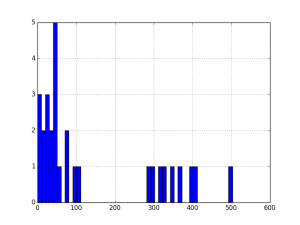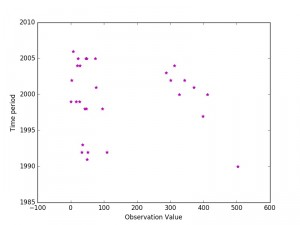```

Data Analysis and Visualization with Python | Set 2

Reference:

If you like GeeksforGeeks and would like to contribute, you can also write an article using write.geeksforgeeks.org or mail your article to review-team@geeksforgeeks.org. See your article appearing on the GeeksforGeeks main page and help other Geeks.